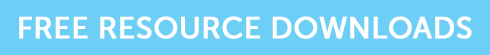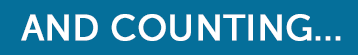Use These Shortcuts to Help with the CII R02 Exam

# Use These Shortcuts to Help with the CII R02 Exam

If you are taking the CII R02 exam and struggling with Chapter 4  (equally helpful for J10 and AF4), then here are a couple of short cuts you may or may not be aware of.

## Question:

Georgia invests £10,000. The investment grows at 5% per annum. How much is it worth after 5 years?

This is a very common exam question. You can, of course, use your scientific calculator – if you have one – but if like a lot of us you just have your old faithful manual one, here are four easy steps to working this through:

Step 1 = enter the interest rate as a decimal plus 1 – so 1.05.

Step 2 = press the multiplication key (once or twice depending on your calculator, you will need to experiment).

Step 3 = enter present value £10,000.

Step 4 = press the = button the number of times that matches the number of time periods.

And if you need to do a discounting calculation:

## Question

Georgia made an investment 5 years ago, which has grown by 5% per annum and is now worth £12,763.  How much did she originally invest?

Step 1 = enter the future value of £12,763 into your calculator.

Step 2 = press (divide) once or twice depending on your calculator (experiment to see what works).

Step 3 = enter interest rate as a decimal plus 1 – i.e. 1.05.

Step 4 = press the = button the number of times that matches the number of time periods i.e. 5.